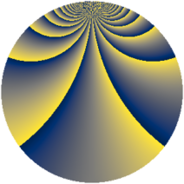# Properties

 Label 3549.2.ciLevel $3549$ Weight $2$ Character orbit 3549.ci Rep. character $\chi_{3549}(274,\cdot)$ Character field $\Q(\zeta_{13})$ Dimension $2160$ Sturm bound $970$

# Related objects

## Defining parameters

 Level: $$N$$ $$=$$ $$3549 = 3 \cdot 7 \cdot 13^{2}$$ Weight: $$k$$ $$=$$ $$2$$ Character orbit: $$[\chi]$$ $$=$$ 3549.ci (of order $$13$$ and degree $$12$$) Character conductor: $$\operatorname{cond}(\chi)$$ $$=$$ $$169$$ Character field: $$\Q(\zeta_{13})$$ Sturm bound: $$970$$

## Dimensions

The following table gives the dimensions of various subspaces of $$M_{2}(3549, [\chi])$$.

Total New Old
Modular forms 5856 2160 3696
Cusp forms 5760 2160 3600
Eisenstein series 96 0 96

## Trace form

 $$2160q + 4q^{2} - 176q^{4} + 16q^{5} + 12q^{8} - 180q^{9} + O(q^{10})$$ $$2160q + 4q^{2} - 176q^{4} + 16q^{5} + 12q^{8} - 180q^{9} + 8q^{10} + 8q^{11} + 16q^{13} + 8q^{15} - 168q^{16} + 40q^{17} + 4q^{18} + 8q^{19} + 80q^{20} - 56q^{22} + 24q^{23} - 132q^{25} + 40q^{26} + 32q^{29} + 8q^{30} - 64q^{31} + 108q^{32} + 8q^{33} - 180q^{34} - 176q^{36} + 16q^{37} + 56q^{38} + 8q^{39} - 92q^{40} + 56q^{41} + 4q^{42} + 32q^{43} + 72q^{44} + 16q^{45} + 56q^{46} + 48q^{47} + 32q^{48} - 180q^{49} + 68q^{50} + 24q^{51} - 172q^{52} - 56q^{53} - 180q^{55} + 36q^{58} + 72q^{59} - 152q^{60} + 48q^{61} - 180q^{62} - 128q^{64} + 76q^{65} - 160q^{66} + 12q^{67} + 104q^{68} + 8q^{69} + 24q^{70} + 48q^{71} + 12q^{72} + 88q^{73} - 28q^{74} + 16q^{77} - 224q^{78} + 104q^{79} + 232q^{80} - 180q^{81} - 212q^{82} + 112q^{83} - 48q^{85} + 80q^{86} - 176q^{87} - 280q^{88} + 144q^{89} + 8q^{90} + 4q^{91} + 120q^{92} - 24q^{94} + 16q^{95} + 40q^{96} + 88q^{97} + 4q^{98} + 8q^{99} + O(q^{100})$$

## Decomposition of $$S_{2}^{\mathrm{new}}(3549, [\chi])$$ into newform subspaces

The newforms in this space have not yet been added to the LMFDB.

## Decomposition of $$S_{2}^{\mathrm{old}}(3549, [\chi])$$ into lower level spaces

$$S_{2}^{\mathrm{old}}(3549, [\chi]) \cong$$ $$S_{2}^{\mathrm{new}}(169, [\chi])$$$$^{\oplus 4}$$$$\oplus$$$$S_{2}^{\mathrm{new}}(507, [\chi])$$$$^{\oplus 2}$$$$\oplus$$$$S_{2}^{\mathrm{new}}(1183, [\chi])$$$$^{\oplus 2}$$